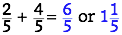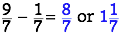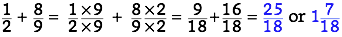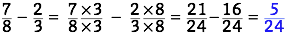# Wearing Uniforms

## OBJECTIVE: To learn how to add and subtract fractions

In a previous lesson, you learned a few terms involving classification of fractions, such as unit fractions, proper fractions, improper fractions, mixed fractions, and equivalent fractions. Cutting Down to Size: https://www.geogebra.org/m/y2v77wpx In this lesson, you'll be introduced to another way of classifying fractions: SIMILAR FRACTIONS (or LIKE FRACTIONS) and DISSIMILAR FRACTIONS (or UNLIKE FRACTIONS). SIMILAR FRACTIONS have the same denominator, whereas DISSIMILAR FRACTIONS have different denominators. The importance of knowing this distinction is for purposes of combining fractions either by addition or by subtraction. The process of ADDING or SUBTRACTING FRACTIONS is easy and straightforward if you're dealing with SIMILAR FRACTIONS. Just add or subtract the numerators and copy the common denominator. Examples:However, adding or subtracting DISSIMILAR FRACTIONS calls for other techniques to perform the needed operation. One such technique is the BUTTERFLY METHOD, illustrated by the applet below. Here are the steps: Step 0: Set up the addition or subtraction problem. Step 1: Cross-multiply the denominator of the second fraction with the numerator of the first fraction. Step 2: Cross-multiply the denominator of the first fraction with the numerator of the second fraction. Step 3: Write down the cross-products above the respective fractions. Step 4. Multiply the denominators together. Step 5. Add or subtract the cross-products and write the sum or difference in the numerator of the new fraction; write the product of the denominators in the denominator of the new fraction. Step 6. Reduce the new fraction to lowest terms, if possible. Step 7. The BUTTERFLY METHOD is now complete. Use the applet below for practice. Click New addition (the technique works for subtraction as well) and move the slider to go through the 7 steps. Repeat as many times as needed to master the concept.

## Applet by Daniel Mentrard

Another common technique for adding or subtracting DISSIMILAR FRACTIONS involves converting one or both fractions to EQUIVALENT FRACTIONS which have a common denominator. The process is similar to reducing fractions to lowest terms, except that instead of dividing both numerator and denominator by the same number, you now have to multiply them by factors that will make the denominators of both fractions equal to the LEAST COMMON DENOMINATOR (a.k.a. LEAST COMMON MULTIPLE). Once the fractions have been rewritten that way, they can be added or subtracted as SIMILAR FRACTIONS. Examples:Below is a set of problems involving adding and subtracting fractions.